CATEGORIES:

# Introduction to Kinematics and Calculus in Physics

1)A physics student from CPS leaves his house and walks along the pavement toward NU. After 5 minutes it starts to rain and he returns home. The following graph shows his distance from the house as a function of time. At which of the points shown is the student’s velocity (a) zero, (b) constant and positive? (c) constant and negative? (d) increasing in magnitude? (e) decreasing in magnitude?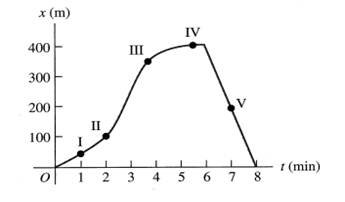2)The figure below shows the qualitative velocity (vx) – time (t) graph of a car that starts from rest at the origin and then accelerates in a straight line x. By referring to this graph, sketch qualitative graphs for(a) the acceleration (ax) – time (t) and

(b) the displacement (x) – time (t).

3)A ball is moving in a straight line along the x-axis. The graph below shows the ball’s velocity as a function of time. (a) What are the ball’s average speed and average velocity during the first 3.00 s? (b) Suppose that the ball moved in such a way so that the graph segment after 2.00 s was -3.00 m/s instead of +3.00 m/s. Find the ball’s average speed and average velocity in this case.4)A rocket is projected vertically up. The positive vertical distance y (in m) it has travelled from the base as a function of time t is given by the equation:

y(t) = (4.60x102)t2 – (16.0) t3

(a) Calculate the average speed of the rocket from t = 0 to t = 10.0 s

(b) Calculate its instantaneous speed at t = 10.0 s

(c) Calculate the maximum height of the rocket

(d) Calculate the maximum speed of the rocket

5)For the following mathematical functions,

a) Find the extrema.

b) Find whether it is maximum or minimum.

c) For each function, plot a graph of y against x showing the positions of extrema.

i)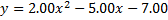ii)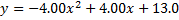6)The position of the front bumper of a test car under crash-testing is given by:

x(t) = 2.17 m + (4.18 ms-2) t2 – (0.100 ms-6) t6

(a) Find the position and acceleration at the instant when the car has zero velocity

(b) Draw x-t, vx-t and ax - t graphs for the motion of the bumper between t = 0 and t = 2.00 sNumerical answers for PSC 03, Kinematics and Calculus in Physics 2014

1) a) IV, b) I, c) V, d) II, e) III

2) Graphs – No Numerical Answers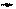3) a) Vave=2.33 ms-1 , Vave=2.33 ms-1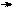b) Vave=0.333 ms-1 , Vave=2.33 ms-1

4) a) 3.00 kms-1

b) 4.40 kms-1

c) 56.3 km

d) 4.41 kms-1

5) a) 1.25 , minima

b) 0.500 , maxima

6) a) 12.6 m , -33.3 ms-2

Date: 2015-01-29; view: 4670

 <== previous page | next page ==> Conclusion. | PSC 38 - Faradays Law 2 with Numerical Answers
doclecture.net - lectures - 2014-2023 year. Copyright infringement or personal data (0.007 sec.)